# Solution assignment 01 of Estimation

1. Annual income presidents
The data for this assignment are listed in the Excel file Annual income presidents.
A survey of 80 randomly selected companies asked them to report the annual income of their presidents. Assume that incomes are normally distributed with a standard deviation of 30,000 dollar.
a. Determine the 90% confidence interval estimate of the annual income of all company presidents. Interpret the statistical results.
b. What would happen to the interval width if we take the 95% confidence interval?
a. The 90% confidence interval estimate is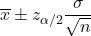.  The sample mean isdollar (computed from the data in the data file), the population standard deviation was given (30,000 dollar), the sample size is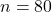and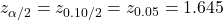. This results in the interval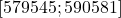. We estimate that the mean annual income of all company presidents lies in this interval; this type of estimate is correct 90% of the time.
b. If the 95% confidence interval estimate was taken then only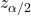changes which becomes larger: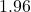. The 95% confidence interval will be wider.
c. Now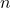will be larger and thus the interval will be narrower.